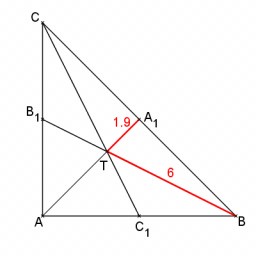# Perpendicular 73574

The two lines of the triangle are perpendicular to each other and are 27 cm and 36 cm. Calculate the length of the sides of the triangle and the length of the third line.

a =  30 cm
b =  51.264 cm
c =  43.2666 cm
t3 =  45 cm

### Step-by-step explanation:

Try calculation via our triangle calculator.Did you find an error or inaccuracy? Feel free to write us. Thank you!

Tips for related online calculators
Do you want to convert time units like minutes to seconds?